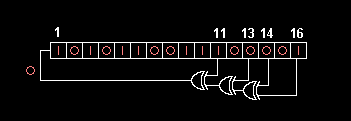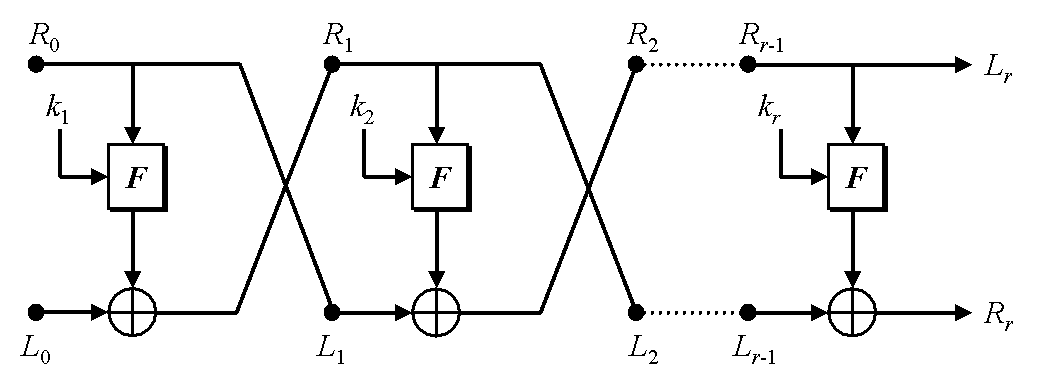# Preliminaries

## Bytes in Python

Python 3 has a literal for a string of bytes:

$python3 >>> type('123\xff') <class 'str'> >>> type(b'123\xff') <class 'bytes'>  Converting array of integers to byte string: $python3
>>> bytes([1, 0xff, 0b0101, 0o177])
b'\x01\xff\x05\x7f'
>>> bytes([0x100])
ValueError: bytes must be in range(0, 256)


Converting byte string to array of integers and applying XOR. When we iterate over a byte string, we get strings of length 1 in Python 2 and integers in Python 3:

$python3 >>> import itertools, operator >>> bytes(itertools.starmap(operator.xor, zip(b'\x01\x02\x03', b'\x02\x03\x04'))) b'\x03\x01\x07'  Displaying bytes in hex or base64: $ python3
>>> import binascii, base64
>>> binascii.hexlify(b'foo bar')
b'666f6f20626172'
>>> binascii.unhexlify(binascii.hexlify(b'foo bar'))
b'foo bar'
>>> base64.b64encode(b'foo bar')
b'Zm9vIGJhcg=='
>>> base64.b64decode(base64.b64encode(b'foo bar'))
b'foo bar'


## Random Bytes

$python2 >>> import os, random >>> b''.join([chr(random.randint(0, 255)) for _ in range(0, 4)]) '\x1d\x07+!' >>> os.urandom(4) 'N\xa4E\xd1'$ python3
>>> bytes([random.randint(0, 255) for _ in range(0, 4)])
b'\xc6xG\xce'
>>> os.urandom(4)
b'\xc7\xe5\x9co'


Definition of cryptographically secure randomness.

## Pseudorandom Generator: PRG

A PRG is a function

(1)
\begin{align} G: \{0, 1\}^n -> \{0, 1\}^* \end{align}

The n bit input is called the random seed. The PRG converts the seed to a string of bits of arbitrary length.

A PRG statistical test is an function from {0, 1}n → {0, 1}. A good test will return 1 for random strings and 0 for non-random strings.

The advantage of a statistical test A against a PRG G is:

(2)
\begin{align} Adv(A, G) := \big| Pr[A(G(k)) = 1] - Pr[A(r) = 1] \big| \end{align}

Here k is randomly drawn from the key space and r is a randomly drawn from {0, 1}n.

If the advantage is close to 1, the statistical test can consistently distinguish the PRG from random text.

A PRG is secure if there are no "efficient" statistical tests with non-negligible advantage. The question of whether there are any secure PRGs depends on whether P = NP. If so, then there are none.

A PRG is secure if and only if there are no "efficient" next bit predictors.

## Linear Feedback Shift Register: LFSR

A linear feedback shift register is a way to implement a PRG in hardware. Here is an example:The example is a 16-bit LFSR, since it has 16 bits of state.

The circuit only uses XOR operations. Are LFSRs ever constructed with other gates?

The circuit uses its state to determine the next bit. The bit is pushed into the 1-bit position, and all other bits are shifted into the next higher position. The 16-bit is discarded from the state.

If we let xi represent the i-th bit, then the next bit is

(3)
\begin{align} ((x_{16} \oplus x_{14}) \oplus x_{13}) \oplus x_{11} = x_{16} \oplus x_{14} \oplus x_{13} \oplus x_{11} \end{align}

If an LFSR has n bits of state, then its period is at most 2n - 1?

Is the period the same for all initial states? No, consider all zeros.

feedback polynomial (feedback function)

## Pseudorandom Function: PRF

For keyspace K and sets X and Y, a pseudorandom function is a function:

(4)
\begin{align} F: K \times X \rightarrow Y \end{align}

That is, for each k ∈ K, we get a function f ∈ Fun[X, Y].

A PRF is secure if for any x ∈ X, F(k, x) is indistinguishable from f(x) for f chosen randomly from F[X, Y].

This can be expressed as a game, in which the adversary chooses x ∈ X, the challenger flips a coin and returns F(k, x) or f(x) for f randomly and uniformly chosen from F[X, Y]. The adversary then tries to guess whether the challenger used F(k, ⋅) or f(⋅).

A secure PRF can be used to create a secure PRG. If F: K × {0, 1}n → {0, 1}n, then we can define G: K × {0, 1}n → {0, 1}nt with:

(5)
\begin{align} G(k) = F(k, 0) \; || \; F(k, 1) \; || \; \ldots \; || \; F(k, t - 1) \end{align}

## Pseudorandom Permutation: PRP

A PRP is a PRF P:K × X → X where p(k, ⋅) is invertible for each k ∈ K.

A PRP is secure if it is a secure PRF.

A Feistel network can be used to construct a PRP from a PRF:In this construction, the PRF F is called the round function. Ruby and Lackoff showed that if F is secure and the Feistel network contains 3 rounds, then the PRP is secure.

Note that the key for the PRP is (k1, k2, k3).

Ruby Lackoff to construction

## Cipher

A triple (𝒦, ℳ, 𝒞) consisting of a key space, a message space and a cipher space, and a pair of efficient algorithms (E, D) where E: (𝒦, ℳ) → 𝒞 and D: (𝒦, 𝒞) → ℳ.

The algorithms must satisfy the consistency equation: D(K, E(K, M)) = M

An efficient algorithm can be taken to be one that runs in polynomial time, but other definitions are used.

# Classical Ciphers

## Substitution Cipher

A substitution cipher is a simple cipher in which each character is mapped to a different character in an injective manner. The key is thus a permutation on the set of characters. If the set of characters is just the upper case English alphabet, then the keyspace has 26! keys.

Some well known ciphers are substitution ciphers with a specific choice of key. With a Caesar cipher, each character is replaced by another character using modular arithmetic. Caesar himself supposedly used a shift by negative 3 to encrypt and a shift by positive 3 to decrypt: that is to encrypt, D was replaced by A, E was replaced by B, and so on. ROT13 is another example of a Caesar cipher with the property that encryption and decryption are the same operation.

Another example of a substitution cipher is the Atbash cipher, used by early Hebrews. The first letter of the alphabet, alef א, was replaced by the last letter of the alphabet, tav ת. The second letter of the alphabet, bet ב, was replaced by the second to last letter of the alphabet, shin ש. The process continues, but the name of the cipher derives from these four letters. The technique can clearly be adapted for the English alphabet, and like ROT13 it has the property the encryption and decryption are the same operation.

A substitution cipher can be implemented with the tr command. First we might need to reduce the set of input characters:

$echo 'ATTACK AT MIDNIGHT!' | tr a-z A-Z | tr -dc A-Z ATTACKATMIDNIGHT  Then encrypt: $ echo ATTACKATMIDNIGHT | tr D-ZA-C A-Z
XQQXZHXQJFAKFDEQ


Reverse the order of the arguments to decrypt:

echo XQQXZHXQJFAKFDEQ | tr A-Z D-ZA-C ATTACKATMIDNIGHT  The Caesar cipher implemented in Python: import re import string rx_not_upper_ascii = re.compile(r'[^A-Z]') lets = string.ascii_uppercase encrypt = string.maketrans(lets[3:] + lets[:3], lets) decrypt = string.maketrans(lets, lets[3:] + lets[:3]) plain_text = rx_not_upper_ascii.sub('', 'Attack at midnight!'.upper()) cipher_text = plain_text.translate(encrypt) plain_text2 = cipher_text.translate(decrypt)  ## Random Key Generation Using a well-known substitution cipher is not cryptographically secure, since an attacker can simply decrypt the cipher text using each of them and see whether any of the resulting plain text messages are sensible. Better would be to choose a key randomly. That is, each possible substitution cipher is equally likely to be chosen. With a 26 character set there are 26! ≈ 288 possible keys, which is a fairly large key space even by modern standards. Selecting a random substitution key is equivalent to shuffling the set of characters. This in turn is equivalent to applying a randomly chosen permutation to the set, where each permutation of appropriate size is equally likely to be chosen. Shuffling a string in Python: import random import string a = list(string.ascii_uppercase) random.shuffle(a) key = ''.join(a) key  ## Substitution Cipher Cryptanalysis cipher text only attack entropy analysis ## Transposition Cipher ## Rotor Machine rotor machine the Hebern machine (1 rotor) and the Enigma (3-5 rotors) Unix version 6 crypt command used a rotor? # Stream Ciphers ## One Time Pad vigenere cipher repeat a key of fixed length to cover the text to be decrypted. Add the key modulo 26 to the message. One Time Pad. Vernam 1917. An encryption scheme (𝒦, ℳ, 𝒞) has perfect secrecy if for any two messages m0 and m1 and any cipher text c: (6) \begin{align} Pr[E(k, m_0) = c] = Pr[E(k, m_1) = c] \end{align} Here the key k is chosen uniformly randomly from 𝒦. Shannon showed that the one time pad has perfect secrecy. He also showed that if an encryption scheme has perfect secrecy, then the key space is as big as or larger than the message space. Two time pad attack. If a one time pad is re-used, the attacker can XOR the two cipher texts to get the xor of the plain texts: m1 XOR m2. Frequency analysis can then be used to recover m1 and m2. HOW TO DO THIS? One time pad lacks integrity. Malleability attack example... ## Stream Cipher Semantic security: a stream cipher which uses a secure PRG is semantically secure. Use a random number or key K of size 2s to generate a pseudorandom number G(K) of size 2n which is long enough to encrypt the message in the manner of a one time pad. Two time pad attacks on stream ciphers. Stream ciphers bad for disk encryption when files are edited. # Block Ciphers ## Block Cipher A function F: K × X → Y is a secure pseudorandom function if F(k, ⋅) cannot be distinguished from f(⋅) where f is a function chosen at random from the entire set of functions from X → Y. This is tested by allowing the attacker to choose x, and then returning F(k, x) or f(x) with equal probability, and seeing if the attacker can distinguish which value was returned. A secure pseudorandom permutation is a secure PRF where X = Y and the function is invertible. A block cipher... ## DES DES 1974 Data Encryption Standard 256 keys, encrypts in 64 bit blocks, can be brute-force attacked today crypt and crack. Password guessing. crypt is DES for passwords; what about DES library for messages. brute force attack on DES. Triple DES... ## AES AES is a block cipher. It always works on 16 bytes (128 bites), though the key can be 16, 24, or 32 bytes (128, 192, or 256 bites). Mathematically, AES is a pseudorandom permutation. It is secure, as far as anyone knows.  sudo pip3 install pycrypto
\$ python3
>>> from Crypto.Cipher import AES
>>> import os
>>> AES.block_size
16
>>> iv = os.urandom(AES.block_size)
>>> iv
b"\xbe-6\x07F\x1e\xbd\x01f\xf6_#\xa0\xf1\x01'"
>>> key = b'Sixteen byte key'
>>> len(key)
16
>>> cipher = AES.new(key, AES.MODE_ECB, iv)
>>> msg = b'Attack at dawn'
>>> padded_msg = msg + bytes(0 for _ in range(0, 16 - len(msg)))
b'Attack at dawn\x00\x00'
>>> ciphertext
b'\xc3L\xfd/\xedD\xd9Y\xfe\x1c\xd8\xc9\x02\x9b\x94\xed'
>>> cipher.decrypt(ciphertext)
b'Attack at dawn\x00\x00'


# Message Integrity

## ECBC-MAC

A PRP F(k, ⋅): K × X → X is needed.

Two keys, k and k1 are needed. Each block is encrypted with F(k, ⋅) and then XOR with the next block before it is encrypted. The final result is then encrypted with F(k1, ⋅) to get the MAC tag.

A diagram showing the process...

A good exercise is to show why, if the final encryption with F(k1, ⋅) is omitted, the authentication scheme is insecure.